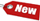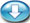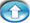Browse Accounting Lessons HereAccounting Terms & DefinitionsAccounting for Merchandising ActivitiesDebits and Credits (Double Entry Accounting)Business Valuation FormulasTime Value of Money & Present/Future ValuesComplex Debt & Equity InstrumentsCommon Stock & Shareholder's EquityAccounting & Finance RatiosValuing Common StockCorporate Income TaxesLower of Cost or Market (LCM) & Inventory ValuationChart of Accounts & BookkeepingBonds Payable & Long Term LiabilitiesCapital AssetsGAAP, Accrual & Cash Accounting, Information Commodity, Internal Controls & MaterialityWhat category of browser are you on this website? Accounting student (homework help) Finance professor (university research) Accounting manager (at work) Other Explore Careers in Accounting and FinanceVisit our section on Careers in Accounting & Finance to explore vast opportunities in this industry.

Chapter 4.6® - Nominal to Effective Interest Rate Calculations & Practice Questions #8 - #16

Question:

10% compounded semi-annually is equivalent to what effective rate?

 i) Press 2nd, and then press 2 ii) NOM = Press 10, Enter theniii) EFF= Pressiv) C/Y = Press 2, then Enter, thenv) EFF = Press CPT vi) Answer = 10.25%

9. Question

7.5% compounded annually is equivalent to what effective rate?

 i) Press 2nd, and then press 2 ii) NOM = Press 7.5, Enter theniii) EFF= Pressiv) C/Y = Press 1, then Enter, thenv) EFF = Press CPT vi) Answer = 7.5%

10. Question

9% compounded monthly is equivalent to what effective rate?

 i) Press 2nd, and then press 2 ii) NOM = Press 9, Enter theniii) EFF= Pressiv) C/Y = Press 12, then Enter, thenv) EFF = Press CPT vi) Answer = 9.38%

11. Question

9% compounded quarterly is equivalent to what effective rate?

 i) Press 2nd, and then press 2 ii) NOM = Press 9, Enter theniii) EFF= Pressiv) C/Y = Press 4, then Enter, thenv) EFF = Press CPT vi) Answer = 9.31%

12. Question

A deposit of \$1,500 grows to \$4262.04 in 7 years. If the interest is compounded quarterly, what is the effective rate?

 N = 7 I/Y = ? PV = \$-1,500 PMT = \$0 FV = \$4,262.04 2ND I/Y P/Y = 1 C/Y = 4 CPT & I/Y = 15.2%

Note that this 15.2% is a nominal interest rate. What is the effective rate?

 i) Press 2nd, and then press 2 ii) NOM = Press 15.2, Enter theniii) EFF= Pressiv) C/Y = Press 4, then Enter, thenv) EFF = Press CPT vi) Answer = 16.09%

13. Question

A deposit of \$1300 earns \$339.45 interest in 3 years. If the interest is compounded monthly, what is the effective rate?

 N = 3 I/Y = ? PV = \$-1,300 PMT = \$0 FV = \$1,639.45 2ND I/Y P/Y = 1 C/Y = 12 CPT & I/Y = 7.76%

Note that this 7.76% is a nominal interest rate. What is the effective rate?

 i) Press 2nd, and then press 2 ii) NOM = Press 7.76, Enter theniii) EFF= Pressiv) C/Y = Press 12, then Enter, thenv) EFF = Press CPT vi) Answer = 8.04%

Present Value Homework Questioni) An investor requires \$25,000 in 7 years and bank offers him 6.76% effective interest rate based on monthly compounding. What amount must he deposit now to achieve his goal?
Note: We recommend you try this problem on your calculator on your own before looking at the solution below, the only way you will learn is if you practice on your own!

 On your BA II plus financial calculator i) Press 2nd, and then press 2 ii) NOM pressiii) EFF= Press 6.76, Enter theniv) C/Y = Press 12, then Enter, thenv) NOM = Press CPT vi) Answer = 6.56%

Now we will use this 6.56% in our present value calculation below:

 N = 7 I/Y = 6.56 PV = ? PMT = \$0 FV = \$25,000 2ND I/Y P/Y = 1 C/Y = 12 CPT & PV = \$15,814.49

Future Value to Determine Number of Periods QuestionAn investor has \$15,000 and wishes it to grow to \$80,000 in the future. If the interest rate is 10% compounded quarterly, how long will this goal take?
Note: We recommend you try this problem on your calculator on your own before looking at the solution below, the only way you will learn is if you practice on your own!

 N = ? I/Y = 10 PV = \$-15,000 PMT = \$0 FV = \$80,000 2ND I/Y P/Y = 1 C/Y = 4 CPT & N = 16.95 periods